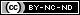# Ordinary Differential EquationsLoading previews...
 Ordinary Differential Equations HTMLViewOrdinary Differential Equations
1 file in this resource
Summary: A differential equation is an equation that contains an unknown function, which we need to solve for, and its derivatives. Technically they are ordinary differential equations (ODEs) since they contain ordinary derivatives as opposed to partial derivatives. An equation that contains partial derivatives is called a partial differential equation (PDE). In this module we shall only consider ordinary differential equations. Differential equations are extremely important in science and engineering as they can mathematically describe physical processes such as current flow in electrical systems, motion of mechanical systems, fluid flow, chemical reactions, population dynamics, the spread of infectious diseases, and many other natural phenomena. DR Calum MacDonald Academic > School of Computing, Engineering and Built Environment > Department of Electrical and Electronic EngineeringAcademic > School of Computing, Engineering and Built Environment > Department of Mechanical Engineering Copyright © Glasgow Caledonian University Ordinary Differential Equations, First Order, Separable ODEs, Linear ODEs, First Order Linear ODEs, The Integrating Factor Method, Second Order, Linear ODEs with Constant Coefficients, Homogeneous Equations, Non-Homogeneous Equations, The Method of Undetermined Coefficients, Non-Homogeneous ODEs with Initial Conditions World MR Joseph Mills 26 Jun 2019 15:40 10 Jul 2020 07:55 https://edshare.gcu.ac.uk/id/eprint/5007View Item